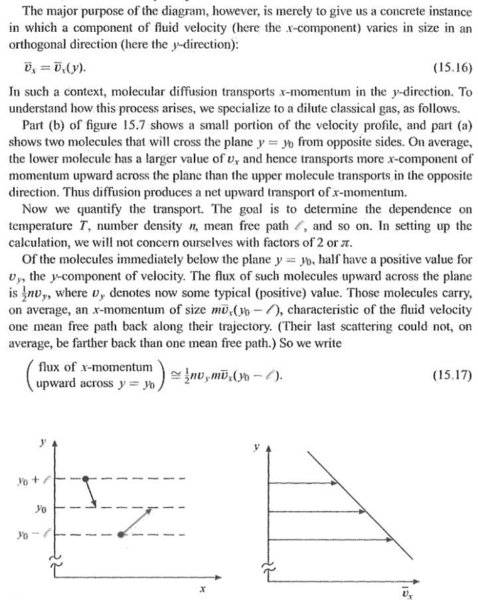# Momentum transport in gases in 2d

• Mohankpvk

#### Mohankpvk

I was trying to understand the momentum transport between gas molecules in 2d.In the image below, it is stated that half of the molecules move up(positive velocity in y direction) and half negative.But the author didnt explain why he assumed it.#### Attachments

Because the whole gas mass is assumed to be static, not moving up, down, left, right.

You could do a different analysis for an upward accelerating gas mass, such as on a rocket, but the answers would change.

•Mohankpvk
anorlunda said:
Because the whole gas mass is assumed to be static, not moving up, down, left, right.

You could do a different analysis for an upward accelerating gas mass, such as on a rocket, but the answers would change.
But why half if the gas is static?Please explain.I referred a few books for momentum transport but all of them used tensors.Is there any book(or other source) in which transport is explained only in 2d?

Mohankpvk said:
But why half if the gas is static?

No, all the particles are moving, some + some -, the average speed of all is zero.

•Mohankpvk

## 1. What is momentum transport in gases in 2D?

Momentum transport in gases in 2D is the movement of momentum (or force) through a gas in a two-dimensional plane. It is a phenomenon that occurs due to the collisions between gas molecules and the walls of a container or between gas molecules themselves.

## 2. How is momentum transported in gases in 2D?

Momentum is transported in gases in 2D through random collisions between gas molecules. These collisions cause the molecules to transfer momentum to each other, resulting in a net movement of momentum through the gas.

## 3. What is the importance of studying momentum transport in gases in 2D?

Studying momentum transport in gases in 2D is important for understanding the behavior of gases in various environments, such as in industrial processes or in planetary atmospheres. It also has applications in fields such as fluid dynamics and heat transfer.

## 4. How is momentum transport in gases in 2D different from 3D?

The main difference between momentum transport in gases in 2D and 3D is the dimensionality of the system. In 2D, the movement of gas molecules is confined to a plane, while in 3D, they can move in all three dimensions. This difference can affect the rate and direction of momentum transport.

## 5. What factors affect momentum transport in gases in 2D?

The factors that can affect momentum transport in gases in 2D include the temperature and pressure of the gas, the size and shape of the container, and the properties of the gas molecules such as their mass and velocity. External forces, such as gravity, can also play a role in momentum transport.